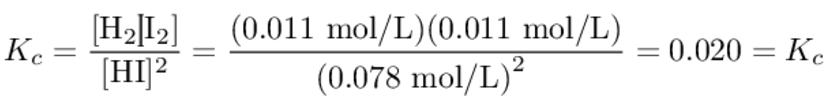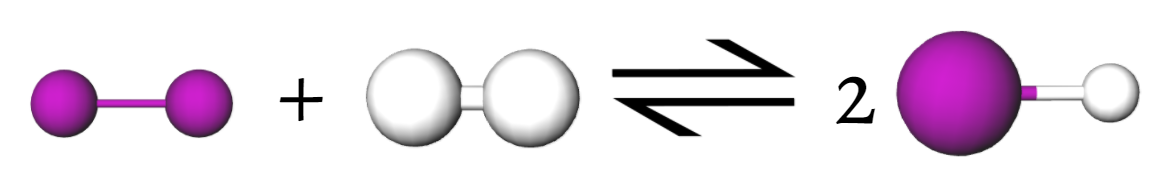# 13.12: Effect of Adding a Reactant or Product

$$\newcommand{\vecs}{\overset { \rightharpoonup} {\mathbf{#1}} }$$ $$\newcommand{\vecd}{\overset{-\!-\!\rightharpoonup}{\vphantom{a}\smash {#1}}}$$$$\newcommand{\id}{\mathrm{id}}$$ $$\newcommand{\Span}{\mathrm{span}}$$ $$\newcommand{\kernel}{\mathrm{null}\,}$$ $$\newcommand{\range}{\mathrm{range}\,}$$ $$\newcommand{\RealPart}{\mathrm{Re}}$$ $$\newcommand{\ImaginaryPart}{\mathrm{Im}}$$ $$\newcommand{\Argument}{\mathrm{Arg}}$$ $$\newcommand{\norm}{\| #1 \|}$$ $$\newcommand{\inner}{\langle #1, #2 \rangle}$$ $$\newcommand{\Span}{\mathrm{span}}$$ $$\newcommand{\id}{\mathrm{id}}$$ $$\newcommand{\Span}{\mathrm{span}}$$ $$\newcommand{\kernel}{\mathrm{null}\,}$$ $$\newcommand{\range}{\mathrm{range}\,}$$ $$\newcommand{\RealPart}{\mathrm{Re}}$$ $$\newcommand{\ImaginaryPart}{\mathrm{Im}}$$ $$\newcommand{\Argument}{\mathrm{Arg}}$$ $$\newcommand{\norm}{\| #1 \|}$$ $$\newcommand{\inner}{\langle #1, #2 \rangle}$$ $$\newcommand{\Span}{\mathrm{span}}$$$$\newcommand{\AA}{\unicode[.8,0]{x212B}}$$

If we have a system which is already in equilibrium, addition of an extra amount of one of the reactants or one of the products throws the system out of equilibrium. Either the forward or the reverse reaction will then occur in order to restore equilibrium conditions. We can easily tell which of these two possibilities will happen from Le Chatelier's principle. If we add more of one of the products, the system will adjust in order to offset the gain in concentration of this component. The reverse reaction will occur to a limited extent so that some of the added product can be consumed. Conversely, if one of the reactants is added, the system will adjust by allowing the forward reaction to occur to some extent. In either case some of the added component will be consumed.

We see this principle in operation in the case of the decomposition of HI at high temperatures:

$\text{2HI}(g) \rightleftharpoons \text{H}_2(g) + \text{I}_2(g) \nonumber$In Example 1 from Calculating the Extent of a Reaction we saw that if 1 mol HI is heated to 745 K in a 10 L flask, some of the HI will decompose, producing an equilibrium mixture of composition 1 $$\text{[HI]} = \text{0.0780 mol/L}$$ ; $$\text{[I}_2] = \text{0.0110 mol/L}$$; $$\text{[H}_2] = \text{0.0110 mol/L}$$ This is a genuine equilibrium mixture since it satisfies the equilibrium lawIf an extra mole of H2 is added to this mixture, the concentrations become 2 $$\text{[HI]} = \text{0.0780 mol/L}$$ ; $$\text{[I}_2] = \text{0.0110 mol/L}$$ ; $$\text{[H}_2] = \text{0.111 mol/L}$$ The system is no longer in equilibrium (hence the lack of square brackets to denote equilibrium concentrations) as we can easily check from the equilibrium lawThe addition of H2 has increased the concentration of this component. Accordingly, Le Chatelier’s principle predicts that the system will achieve a new equilibrium in such a way as to reduce this concentration. The reverse reaction occurs to a limited extent. This not only reduces the concentration of H2 but the concentration of I2 as well. At the same time the concentration of HI is increased. The system finally ends up with the concentrations calculated in Example 2 from Calculating the Extent of a Reaction, namely,

$\text{H}_2+ \text{I}_2 \rightarrow \text{2HI} \nonumber$3 $$\text{[HI]} = \text{0.0963 mol/L}$$ ;$$\text{[I}_2] = \text{0.001 82 mol/L}$$ ; $$\text{[H}_2] = \text{0.1018 mol/L}$$ This is again an equilibrium situation since it conforms to the equilibrium law

$\frac{\text{ }\!\![\!\!\text{ H}_{\text{2}}]\text{ }\!\!]\!\!\text{ }\!\![\!\!\text{ I}_{\text{2}}\text{ }\!\!]\!\!\text{ }}{\text{ }\!\![\!\!\text{ HI }\!\!]\!\!\text{ }^{\text{2}}}=\frac{\text{0}\text{.1018 mol L}^{-\text{1}}\text{ }\times \text{ 0}\text{.001 82 mol L}^{-\text{1}}}{\text{(0}\text{.0963 mol L}^{-\text{1}}\text{)}^{\text{2}}\text{ }}=\text{0}\text{.02}=K_{c} \nonumber$

The way in which this system responds to the addition of H2 is also illustrated schematically in Figure 1. The actual extent of the change is exaggerated in this figure for diagrammatic effect.Figure $$\PageIndex{1}$$ Le Chatelier’s principle: effect of adding a component. At 745 K, HI is partially decomposed into H2 and I2: 2HI H2 + I2. If extra hydrogen (gray) is added to the equilibrium mixture, the system responds in such a way as to reduce the concentration of H2. Some I2 reacts with the H2, and more HI is formed. The equilibrium is shifted to the left. Note, however, that some of the I2 has been consumed, and its concentration is smaller than before.

Le Chatelier's principle can also be applied to cases where one of the components is removed. In such a case the system responds by producing more of the component removed. Consider, for example, the ionization of the weak diprotic acid H2S:

$\ce{H_{2}S + 2H_{2}O \rightleftharpoons 2H_{3}O^{+} + S^{2-}} \nonumber$Since H2S is a weak acid, very few S2– ions are produced, but a much larger concentration of S2–ions can be obtained by adding a strong base. The base will consume most of the H3O+ ions. As a result, more H2S will react with H2O in order to make up the deficiency of H3O+, and more S2–ions will also be produced. This trick of removing one of the products in order to increase the concentration of another product is often used by chemists, and also by living systems.

Previously, we've investigated the effect of adding/subtracting a product/reactant in mathematical terms. The video below allows you to visually see the changes the equilibrium shifts that occur upon the addition of a reactant or product, courtesy of the North Caroline School of Science and Mathematics.

##### Example $$\PageIndex{1}$$ : Yield

When a mixture of 1 mol N2 and 3 mol H2 is brought to equilibrium over a catalyst at 773 K (500°C) and 10 atm (1.01 MPa), the mixture reacts to form NH3 according to the equation

$$\text{N}_2(g) + \text{3H}_2(g) \rightleftharpoons \text{2NH}_3(g)$$ ΔHm = – 94.3 kJ The yield of NH3, however, is quite small; only about 2.5 percent of the reactants are converted. Suggest how this yield could be improved (a) by altering the pressure; (b) by altering the temperature; (c) by removing a component; (d) by finding a better catalyst.

Solution:

a) Increasing the pressure will drive the reaction in the direction of fewer molecules. Since Δn = - 2, the forward reaction will be encouraged, increasing the yield of NH3. b) Increasing the temperature will drive the reaction in an endothermic direction, in this case in the reverse direction. In order to increase the yield, therefore, we need to lower the temperature. c) Removing the product NH3 will shift the reaction to the right. This is usually done by cooling the reaction mixture so that NH3(l) condenses out. Then more N2(g) and H2(g) are added, and the reaction mixture is recycled to a condition of sufficiently high temperature that the rate becomes appreciable. d) While a better catalyst would speed up the attainment of equilibrium, it would not affect the position of equilibrium. It would therefore have no effect on the yield.

Note: As mentioned in Chaps. 3 and 12, NH3 is an important chemical because of its use in fertilizers. In the design of a Haber-process plant to manufacture ammonia, attempts are made to use as high a pressure and as low a temperature as possible. The pressure is usually of the order of 150 atm (15 MPa), while the temperature is not usually below 750 K. Although a lower temperature would give a higher yield, the reaction would go too slowly to be economical, at least with present-day catalysts. The discoverer of a better catalyst for this reaction would certainly become a millionaire over-night.